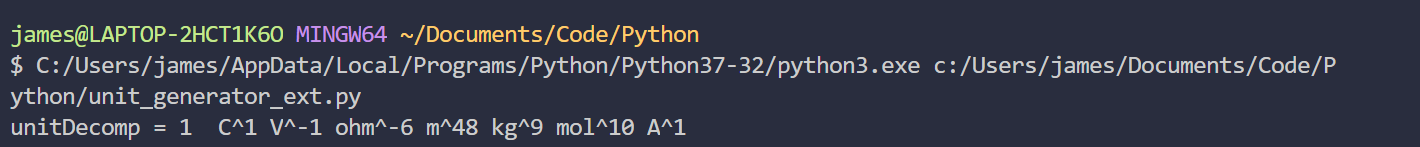I’ve recently had the idea to build a calculator that can take a unit, like seconds, and obfuscate it to some arbitrary level by multiplying and dividing by a stack of different units. Since we’re just multiplying and dividing by units that will, eventually, cancel, we can also take each unit to an arbitrary positive or negative power (though I think fractional exponents are probably likely to cause some interesting problems with display).

Here’s an example.### Motivation

I like the ideas of abuse of notation, units and dimensional analysis (Relevant XKCD), but coming up with complicated equivalent expressions for simple value/unit combinations is tedious at best. Why not, then, automate this with a program, that lets us create as an as arbitrarly complicated equivalent expression as we want?

## The Algorithm

1) Take in an SI unit for the equivalent expression (Extension: Take in a combination of units, multiplied or divided, and a value)
2) Randomly select other SI-derived units (like Teslas, Pascals or Coulombs) from a list of units, and write the the unit symbol (eg. Pascal = Pa) to another array.
3) For the selected units, raise them to an arbitrary power, and add the value of the power to the appropriate element in an array whose entries signify the power of each unit.
4) Once we’re done iterating through the SI-Derived units array, we’ll have a complex expression that will not be equivalent to the input unit. To offset this we have another array - the SI equivalent array
5) This array has a length of 7, and each entry gives the equivalent power of each SI unit. For example, we’ll have [1, 0, 6, 0, 2, 31, 0], for the equivalent powers of [kg, mol, s, Cd, A, K, m], suggesting that our random selection of SI-derived units is equivalent to:

$latex kg \cdot s^6 \cdot A^2 \cdot K^31 &s=2$

6) To get the numbers in our SI-equivalent array, every time we add a unit to the Si-Derived array, we’ll add the SI-equivalent (-ve exponents for denominator values) to a respective entry. For example, since a Tesla is equivalent to seconds squared times amperes over kilograms, our SI-Equivalent array is updated with [kg-1, mol, s+2, Cd, A+1, K, m].
7) If we can multiply what we’re adding to the SI-Equivalent array by the power of the SI-Derived unit - since a tesla squared would be seconds to the 4th power times amperes squared over kilograms squared, we can add instead [kg-1_2, mol, s+2_2, Cd, A+12, K, m].

8) Now, if we were to divide our our SI-Derived array by our SI-Equivalent array, they’d end up cancelling completely and give us no units. Another way of doing this is multiplying by the inverse (1/X) of the array, which by our system conveniently allows us to take each power and multiply it by -1 ([kg*-1, mol*-1, s*-1, Cd*-1, A*-1, K*-1, m*-1]) to allow us to cancel everything.
9) We can multiply this inverse array by the input unit, so we cancel everything except what the input is, allowing us to get our final result as every unit cancelling except the input unit, which is a nice output. (I don’t have this implemented yet, but it’s easy enough)
10) Finally, we can display every unit with a nonzero power by printing it (unit + “ ^ ” + power) to the output stream.

## A Partial Implementation

 _#abuse of units_

_import_ random

class unit:
def \_\_init\_\_(self,symb, siDecomp, index):
self.symb = symb _#the name of the unit_
self.si = siDecomp _#the SI decomposition, in array form_
self.idx = index

len  = 0 _#metre 1_
time = 0 _#sec 2_
mass = 0 _#kilo 3_
temp = 0 _#kelvin 4_
qty =  0 _#mole 5_
cur =  0 _#amp, coulumb/sec 6_

_#          nulL 1  2    3    4    5   6_
siArray = $0, len,time,mass,temp,qty,cur$ _#could have populated with zeroes_
_#                C  V  ohm  T_
_# nonSIArray_
C = unit("C", $6,1$, 1)
V = unit("V", $3,1,1, -2,-2,-2,-6$,2)
Ohm = unit("ohm", $3, 3, -2,-2,-2,-6, -6$,3)
T = unit("T", $3,-2,-2,-6$,4)

nonSIArray = $C,V,Ohm,T$

randomUnitFill = 
randomUnitBuffStr = $"","","",""$
randomUnitBuffNum = $0, 0, 0, 0$

_#numUnits = input("Number of units to generate")_
numUnits = 20

_if_(numUnits >0):
_for_ i in range(4):
rTimes =random.randint(0,numUnits/2)
_if_ (rTimes!=0):
randomUnitBuffStr$i$ = (nonSIArray$i$.symb)

_for_ j in range(rTimes):
randomUnitBuffNum$i$+=random.choice($1,-1$)
randomUnitFill.append(nonSIArray$i$) _#repeated increase"",_

_for_ unit in randomUnitFill:
_for_ si in unit.si:
siArray$si$+=1;

_# for si in V.si:_
_#     siArray$abs(si)$+= si; #add signed power_

print("unitDecomp = 1 ", end = ' ')

_#nonSI Print_
_for_ i in range (4):
_if_ (randomUnitBuffNum$i$!=0):
print(randomUnitBuffStr$i$+"^"+str(randomUnitBuffNum$i$), end = " ")

_#SI Print_
_for_ i in range (7):
_if_ (siArray$i$!=0):
_if_ (i==1):
print("m", end = "")
_elif_ (i==2):
print ("s", end = "")
_elif_ (i==3):
print ("kg", end = "")
_elif_ (i==4):
print ("K", end = "")
_elif_ (i==5):
print ("mol", end = "")
_elif_ (i==6):
print ("A", end = "")

print("^"+ str(int(siArray$i$/i)), end = ' ') _#gets powers, works for -ves_


## Conclusion and Next StepsSo there are some minor problems with this - it looks ugly and there are some problems errors with the array indexing, as I shouldn’t be getting mols as output anywhere. It’s a work in progress. Plus, I wrote it in an hour, and I haven’t used Python in quite a while. It was definitely harder than I thought to do mathematical manipulations on strings as if they were numbers. My idea to encode the strings themselves as numbers and then map them back was a bit tedious, and perhaps some more advanced datastructures would simplify this problem (maybe a dictionary or some custom objects, with predefined algebra methods).

I think that if I had the time, this would be a pretty interesting WebApp to make, if only to practice writing Javascript. I definitely need to make some improvements to the algorithm, as I have to code each unit by hand and I can imagine that this would take a rather excessive amount of time and code.

If you got all the way to the end, thanks for reading my article. If you have any ideas for things you want to see or suggestions, let me know in the comments section or contact me directly. I normally don’t publish pure programming/algorithms material, but I thought this was an interesting enough idea/half-baked implementation to share. I hope you enjoyed it.